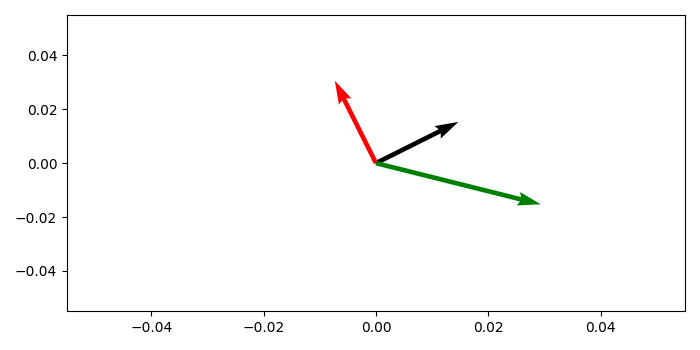# How to plot vectors in Python using Matplotlib?

To plot vectors in Python using matplotlib, we can take the following steps −

• Create a matrix of 2×3 dimension.

• Create an origin point, from where vecors could be originated.

• Plot a 3D fields of arrows using quiver() method with origin, data, colors and scale=15.

## Example

import numpy as np
from matplotlib import pyplot as plt
plt.rcParams["figure.figsize"] = [7.00, 3.50]
plt.rcParams["figure.autolayout"] = True
data = np.array([[2, 1], [-1, 2], [4, -1]])
origin = np.array([[0, 0, 0], [0, 0, 0]])
plt.quiver(*origin, data[:, 0], data[:, 1], color=['black', 'red', 'green'], scale=15)
plt.show()

## Output